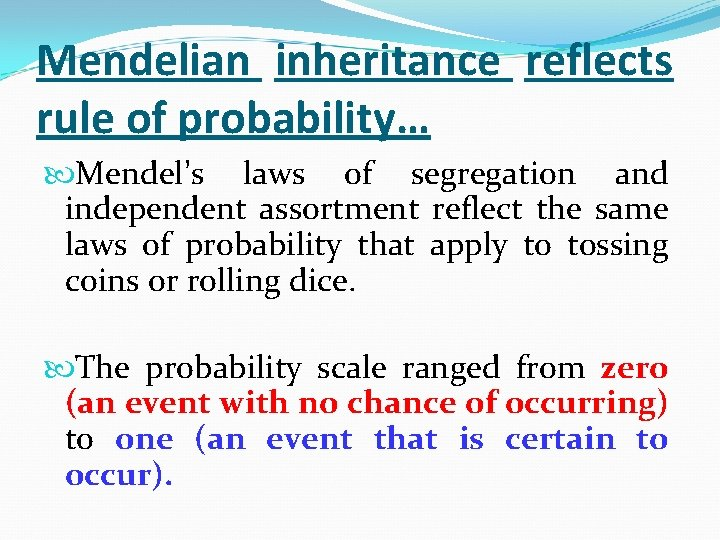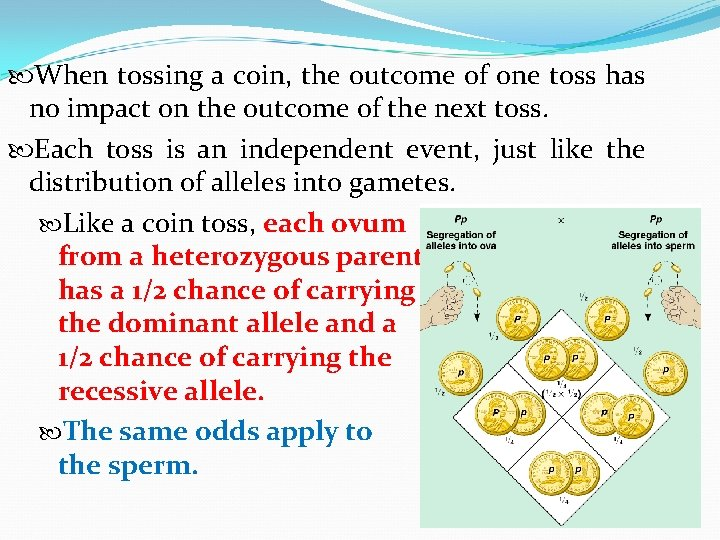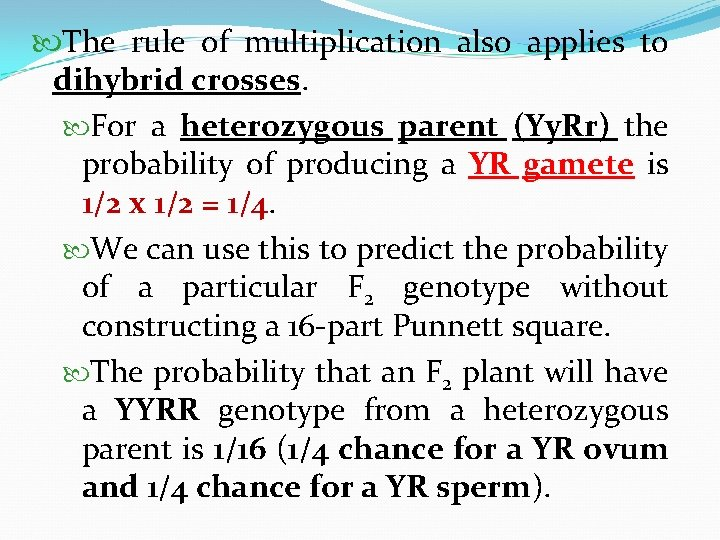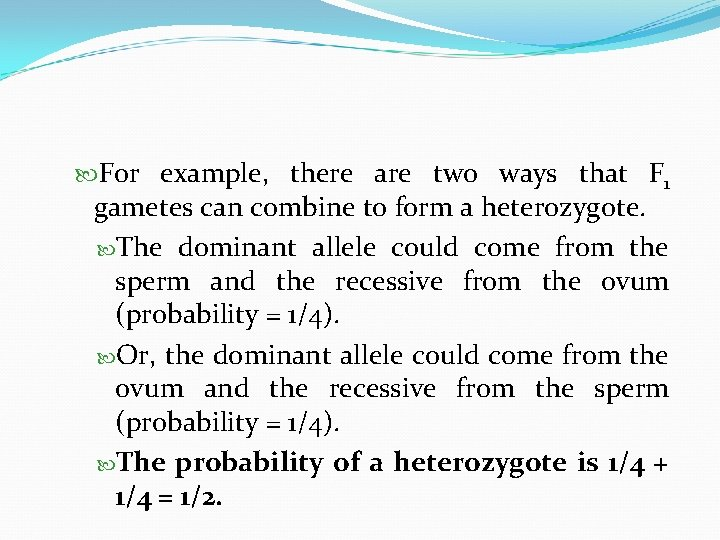# PROBABILITY AND MENDELIAN INHERITANCE Mendelian inheritance reflects rule

• Slides: 10PROBABILITY AND MENDELIAN INHERITANCEMendelian inheritance reflects rule of probability… Mendel’s laws of segregation and independent assortment reflect the same laws of probability that apply to tossing coins or rolling dice. The probability scale ranged from zero (an event with no chance of occurring) to one (an event that is certain to occur).Tossing a coin The probability of tossing heads with a normal coin is 1/2. AND The probability of tossing tails with a normal coin is 1/2.Rolling a Dice The probability of rolling a 3 with a six-sided die is 1/6 And The probability of rolling any other number is 1 - 1/6 = 5/6.When tossing a coin, the outcome of one toss has no impact on the outcome of the next toss. Each toss is an independent event, just like the distribution of alleles into gametes. Like a coin toss, each ovum from a heterozygous parent has a 1/2 chance of carrying the dominant allele and a 1/2 chance of carrying the recessive allele. The same odds apply to the sperm.Rule of Multiplication When to use it: When you want to determine the probability that two or more independent events will occur together in some specific combination. How to use it: Compute the probability of each independent event. Then, multiply the individual probabilities to obtain the overall probability of these events occurring together.EXAMPLE: The probability that two coins tossed at the same time will land heads up is 1/2 x 1/2 = 1/4. Similarly, the probability that a heterozygous pea plant (Pp) will produce a white-flowered offspring (pp) depends on an ovum with a white allele mating with a sperm with a white allele. This probability is 1/2 x 1/2 = 1/4.The rule of multiplication also applies to dihybrid crosses. For a heterozygous parent (Yy. Rr) the probability of producing a YR gamete is 1/2 x 1/2 = 1/4. We can use this to predict the probability of a particular F 2 genotype without constructing a 16 -part Punnett square. The probability that an F 2 plant will have a YYRR genotype from a heterozygous parent is 1/16 (1/4 chance for a YR ovum and 1/4 chance for a YR sperm).Rule of Addition The rule of addition also applies to genetic problems. Under the rule of addition, the probability of an event that can occur two or more different ways is the sum of the separate probabilities of those ways.For example, there are two ways that F 1 gametes can combine to form a heterozygote. The dominant allele could come from the sperm and the recessive from the ovum (probability = 1/4). Or, the dominant allele could come from the ovum and the recessive from the sperm (probability = 1/4). The probability of a heterozygote is 1/4 + 1/4 = 1/2.